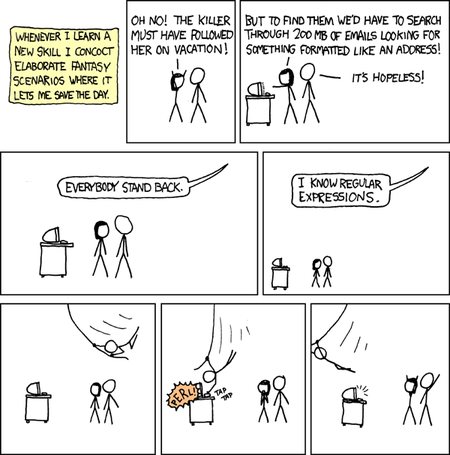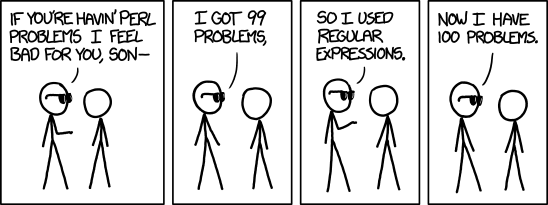##• A ‘language’ to represent text patterns - concept invented in the 1950’s

• Bound by a set of rules (syntax); a set of special characters used to denote patterns

• Multi-platform: available (natively or through libraries) in many languages and tools (R, Python, Java, sed, awk)

• Use cases:

• read files with a specific naming pattern, e.g. 20190114_Mon_P1_W08_R2.csv, 20190114_Mon_P10_W01_R3.csv
• search for text patterns
• replace text patterns

## The basics

• Character classes `[]`

• any character: `.`
• alphabet: `[A-Z]` or `[:upper:]`, `[a-z]` or `[:lower:]`, `[A-Za-z]` or `[:alpha:]`
• numeric: `[0-9]` or `[:digit:]` or `\d`
• alphanumeric: `[A-Za-z0-9]` or `[:alnum:]`
• whitespace (space, tab, linebreak): `\s`
• quantifiers:

• one or more (of the preceding character): `+`
• zero or more: `*`
• zero or one: `?`
• specified number: `{m,}`, `{m,n}`

## The basics

• anchors:

• start: `^` (except in the context of `[^ ]`, where it is negation)
• end: `\$`
• capture groups:

• extract groups: `()`
• refer to captured groups: `\1`, `\2`, etc.
• metacharacters: `. \ | ( ) [ { ^ \$ * + ? ,`

## Examples

• in the string “the cat in the hat has a bat”:

• `[ch]at` matches `cat` and `hat`
• `.at` matches `cat`, `hat` and `bat`
• `.{2}` matches `in`
• `[:alpha:]{1,2}` matches `in` and `a`
• `.\s.` matches `e c`, `t i`, `n t`, `e h`, `t h`, `s a`, `a b`

## Strings in R

• Strings (“character” class) are represented in R using " or ’
• But what about special characters like newlines and tabs? They are represented as escape sequences.`print` prints the escape sequence, whereas `cat` processes them.
``````string = "First\tline\nSecond\tline"
print(string)

##  "First\tline\nSecond\tline"

cat(string)

## First    line
## Second   line
``````

## Strings in R

• What if the string contains an invalid escape character?
``````regex_string = ".\s."

## Error: '\s' is an unrecognized escape in character string starting "".\s"
``````
• Regular expressions are represented as strings in R. But strings are processed first for escape characters. Unrecognised escape characters in strings throw an error, before even reaching the regex parser.
• Double backslahes needed for regex escape sequences
``````regex_string = ".\\s."
string = "the cat in the hat has a bat"
regexpr(regex_string, string)
``````

 3 attr(,“match.length”)  3 attr(,“index.type”)  “chars” attr(,“useBytes”)  TRUE

## Two problems Some people, when

confronted with a problem, think “I know, I’ll use regular expressions.” Now they have two problems. - Jamie Zawinski## Base R functions that use regex

• `grep()`

• `grepl()`

• `regexpr()`

• `gregexpr()`

• `sub()`

• `gsub()`

• `strsplit()`

• `list.files()`

## Stringr functions

As with other Tidyverse functions, Stringr functions take the text as the first argument and the pattern as the second argument

• `str_locate()` - like `regexpr()`, but returns an integer matrix
• `str_detect()` - like `grepl()`
• `str_split()` - like `strsplit()`
• `str_extract()` - like `match = regexpr(pattern, string); substring(string, match, match + attr(match, "match.length") - 1)`

## Test drive

``````library(babynames)
library(stringr)
library(dplyr)
library(ggplot2)
library(ggpubr)
babynames %>%
group_by(name, sex) %>%
summarise(n = sum(n)) -> sum_babynames
``````
``````sum_babynames %>%
filter(str_detect(name, "a\$")) %>%
group_by(sex) %>%
count() %>%
ggplot(aes(x = sex, y = n, fill = sex)) +
geom_col(colour = "black") +
labs(title = "Names ending with 'a'") +
theme_pubr(border = TRUE)
``````

##``````babynames %>%
filter(str_detect(name, "a\$")) %>%
group_by(sex, year) %>%
count() %>%
ggplot(aes(x = year, y = n, colour = sex)) +
geom_line() +
labs(title = "Names ending with 'a'") +
theme_pubr(border = TRUE)
``````

##``````sum_babynames %>%
filter(str_detect(name, "[aeiou]\$")) %>%
group_by(sex) %>%
count() %>%
ggplot(aes(x = sex, y = n, fill = sex)) +
geom_col(colour = "black") +
labs(title = "Names ending with vowel") +
theme_pubr(border = TRUE)
``````

##``````babynames %>%
filter(str_detect(name, "[eiou]\$")) %>%
group_by(sex, year) %>%
count() %>%
ggplot(aes(x = year, y = n, colour = sex)) +
geom_line() +
labs(title = "Names ending with a vowel other than 'a'") +
theme_pubr(border = TRUE)
``````

##``````sum_babynames %>%
filter(str_detect(name, "(.{2})\\1")) %>%
group_by(sex) %>%
#filter(sex == "M") %>%
count() %>%
#print()
ggplot(aes(x = sex, y = n, fill = sex)) +
geom_col(colour = "black") +
labs(title = "Names with repetitive characters") +
theme_pubr(border = TRUE)
``````

##``````sum_babynames %>%
filter(str_detect(name, "[HhZz]ero")) %>%
group_by(sex) %>%
#filter(sex == "M") %>%
#count() %>%
print()

## # A tibble: 36 x 3
## # Groups:   sex 
##    name     sex       n
##    <chr>    <chr> <int>
##  1 Acheron  M        56
##  2 Cherod   M         6
##  3 Cherokee F      2414
##  4 Cherokee M       337
##  5 Cherol   F        17
##  6 Cherolyn F        60
##  7 Cheron   F       635
##  8 Cheron   M        99
##  9 Cheronda F       164
## 10 Cherone  F         7
## # … with 26 more rows
``````
``````babynames %>% group_by(year) %>% count() -> babynames_pop
left_join(babynames, babynames_pop, by = "year") -> babynames_complete

babynames_complete %>%
filter(str_detect(name, "^[^AEIOUaeiou]+\$")) %>%
group_by(sex, year) %>%
summarise(n = sum(n.x/n.y)) %>%
#count() %>%
ggplot(aes(x = year, y = n, colour = sex)) +
geom_line() +
labs(title = "Names without any vowels") +
theme_pubr(border = TRUE)
``````

##``````babynames %>%
filter(str_detect(name, "^Joshua\$")) %>%
group_by(sex, year) %>%